This post introduces more Neural Networks concepts, which can be used to improve the classification of digits from the MNIST dataset.

In this post, you'll learn how to:

• augment image data with affine transforms;
• normalise inputs;
• use activation functions;
• use dropout;
• train and evaluate a Convolutional Neural Network.

By the end, you'll learn how to significantly improve the prediction accuracy compared to the last post: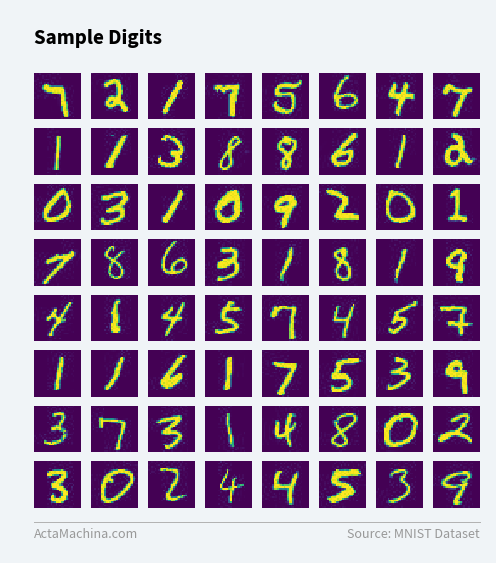## Import Packages¶

We'll be using the PyTorch package from Facebook, which we introduced in the previous post, to build and train Convolutional Neural Networks (CNNs).

In :
from torchvision import datasets, transforms
from torchvision.utils import make_grid

from torch.utils.data.dataset import random_split

import torch
import torch.nn as nn
import torch.nn.functional as F
import torch.optim as optim


## Convolutional Neural Networks¶

### Convolutions¶

A convolution matrix is produced by aligning the top-left corners of a smaller matrix (kernel) and a larger matrix and then multiplying each overlapping element and adding them up. The smaller matrix is then slid one position to the right and the calculation is repeated until you have all the values for the entire row. Then, repeat the same calculations for each row. To think of it in another way, imagine scanning a page with a magnifying glass and summarising only what can be seen through the magnifying glass at each step. The same procedure can be extended to a 3 dimensions, where the kernel has a depth dimension too, operating against an input with the same depth dimension. The dot product is applied to all the elements across all the dimensions.

The intuition behind using convolutions in Neural Networks when dealing with images is that pixels from the same region are more likely to be related compared to pixels that are far away from each other. For this reason, it's often unnecessary and a waste of resources to only use layers that are fully connected, when convolutions, which require a smaller number of weights to be optimised, does the same job.

Out: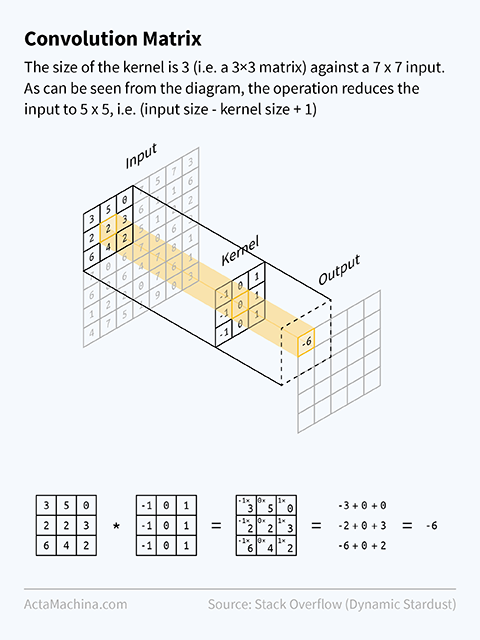Each kernel looks for a particular pattern as dictated by its weights. So, to detect multiple patterns, different kernels can be used to produce a series of convolution matrices. For example, the figure below shows examples of kernel weights that can produce a sharpened image, a blurred image, and an image that has vertical edges enhanced. Here, the kernel size is 5. Out of these three examples, probably only the last kernel will be used by a Convolutional Neural Network as a vertical edge detector.

Out: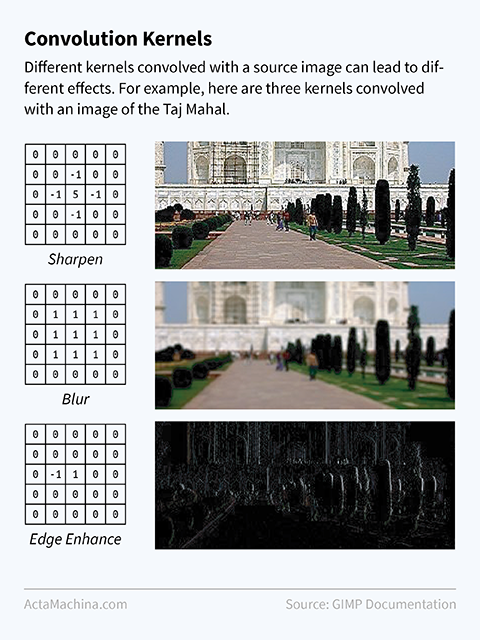Generally, most Convolutional Neural Network architectures apply several kernels simultaneously (called channels) to the previous layer, and as the network gets deeper, an increasing number of channels can be used on successive layers. In the diagram below, the number of channels is increased from 20 to 50, indicated by the depth of the layers. The kernel size will largely remain the same. As the network gets deeper, this results in a network that extracts a larger number of more complicated features. Max Pooling in between convolution layers, which keeps the largest value in a segment, help summarise features for a more general representation of the previous layer (see next section).

The Convolutional Neural Network architecture we'll use for training and predicting digits is:

Out: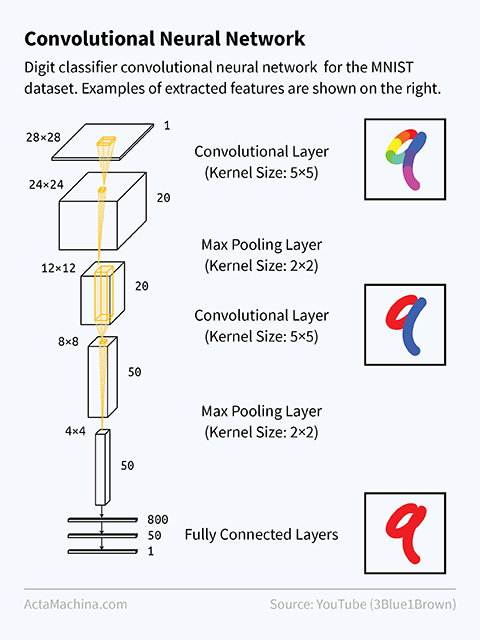## Convolutional Neural Networks with PyTorch¶

To accelerate training and evaluation of the network, we'll be making use of GPUs as covered in the Bonus section of the previous post.

First, we set device to either the CPU or GPU, depending on whether we have access to a GPU:

In :
device = torch.device('cuda:0' if torch.cuda.is_available() else 'cpu')


Now, let's use the Convolutional Neural Network architecture suggested in the previous section and translate it into PyTorch code:

In :
class Net(nn.Module):
def __init__(self):
super(Net, self).__init__()
self.conv1 = nn.Conv2d(1, 20, 5)
self.conv2 = nn.Conv2d(20, 50, 5)
self.dropout = nn.Dropout(p=0.5)
self.fc1 = nn.Linear(50*4*4, 500)
self.fc2 = nn.Linear(500, 10)
self.max_pool = nn.MaxPool2d(kernel_size=2)

def forward(self, x):
x = x                      #  1 x  28 x 28 (original image)
x = F.relu(self.conv1(x))  # 20 x  24 x 24
x = self.max_pool(x)       # 20 x  12 x 12
x = F.relu(self.conv2(x))  # 50 x   8 x  8
x = self.max_pool(x)       # 50 x   4 x  4
x = x.view(-1, 50*4*4)     #  1 x 800      (flatten)
x = F.relu(self.fc1(x))    #  1 x 500
x = self.dropout(x)        #  1 x 500
x = self.fc2(x)            #  1 x 10
return F.log_softmax(x, dim=1)

net = Net().to(device)


Conv2D is the basic building block of the Convolutional Neural Network, which usually make up the earlier layers. The later layers are often Linear, fully-connected layers. Note that we use a kernel size of 5, meaning that we slide a 5 pixel by 5 pixel window across the original image to obtain the convolution matrix.

We'll also be using some other techniques to improve the network such as F.relu(), nn.Dropout(), nn.Linear() and nn.MaxPool2D(). Let's step through them one-by-one and see what how they can improve the network:

• F.relu: ReLu (short for rectified linear unit), is an activation function. This means that the output of the neuron is only "activated" for a set of certain consecutive values, and is inactive (set to zero) for the other values. A neural network without activations can only fit linear combinations of the previous layer and is limited in the functions it can model. Neural Networks that use activation functions can fit non-linear functions making it more flexible. In fact, the Universal Approximation Theorem states that Neural Networks with activation functions can approximate any function if it is deep enough or wide enough.

• nn.Dropout: Any layer with Dropout applied will randomly set the values of its neurons to zero. A dropout probability value of 0.5 means that each neuron has a 50% chance of being set to zero. This uncertainty ensures that the network can't depend on any given neurons too much and helps prevent overfitting of the network.

• nn.Linear: Notice that in the previous post, we set bias=False, but here, we've omitted the parameter. Turning on the bias allows each neuron to access an always-on input and allows the neuron to shift the activation function depending on the weight assigned to the bias.

• nn.MaxPool2D: Max Pooling divides the image into non-overlapping segments and only keeps the largest value in each segment. In this case, since our kernel size is set to 2, the size of each segment is 2 x 2 pixels, and Max Pooling reduces 4 pixels to a single pixel. Max Pooling helps the network generalise by reducing the spatial representation of the image, ensuring that features that are off by a few pixels still end up with the same output.

Tip: Most functions have both a F.* (functional) and a nn.* (module) version, e.g. F.dropout/nn.Dropout(). The difference is that the functional version doesn't store any parameters so we usually reserve it for functions without parameters, while the module version is used for functions with parameters since we only have to declare and process the parameters once.

The dataset and dataloaders are similar to the previous post:

In :
transform = transforms.Compose([
transforms.Grayscale(),
transforms.RandomAffine(degrees=2, translate=(.1, .1), scale=(.9, 1.1), shear=2),
transforms.ToTensor(),
transforms.Normalize((0.1307,), (0.3081,)),
])

dataset = datasets.ImageFolder('data/mnist', transform=transform)

train_dataset, test_dataset = random_split(dataset, [len(dataset)-10000, 10000])



The only exception is that we add two additional transforms, RandomAffine and Normalize:

• RandomAffine is a transform that augments the dataset. Every image in the training set is randomly rotated, translated, scaled and sheared. In the case of the arguments used above, every digit is randomly rotated between -2 and 2 degrees, translated by 10% in both directions, scaled between 90% and 110% and sheared at an angle of -2 and 2 degrees. Each time data is loaded via the Dataloader, these transforms are randomly applied, meaning that to actually increase the number of images in the training set, we also need to increase the number of epochs trained.
• Normalize is a transform that normalises train and test images according to a given mean and standard deviation. The mean and standard deviation for the MNIST dataset is known to be 0.1307 and 0.3081 respectively.

The criterion, optimizer and train/evaluation phases are left untouched:

In :
criterion = nn.CrossEntropyLoss()
optimizer = optim.SGD(net.parameters(), lr=0.001, momentum=0.9)

In [ ]:
net.train()  # set network to training phase

epochs = 25

# for each pass of the training dataset
for epoch in range(epochs):
train_loss, train_correct, train_total = 0, 0, 0

# for each batch of training examples
for batch_index, (inputs, labels) in enumerate(train_dataloader):
inputs, labels = inputs.to(device), labels.to(device)
outputs = net(inputs)  # forward pass
loss = criterion(outputs, labels)  # compare output with ground truth
loss.backward()  # backpropagation
optimizer.step()  # update network weights

# record statistics
_, preds = torch.max(outputs.data, 1)
train_loss += loss.item()
train_correct += (preds == labels).sum().item()
train_total += len(labels)

# print statistics every 100 batches
if (batch_index + 1) % 100 == 0:
print(f'Epoch {epoch + 1}, ' +
f'Batch {batch_index + 1}, ' +
f'Train Loss: {(train_loss/100):.5f}, ' +
f'Train Accuracy: {(train_correct/train_total):.5f}')

train_loss, train_correct, train_total = 0, 0, 0

In :
net.eval()  # set network to evaluation phase

test_loss = 0
test_correct = 0

# for each batch of testing examples
for batch_index, (inputs, labels) in enumerate(test_dataloader):
inputs, labels = inputs.to(device), labels.to(device)
outputs = net(inputs)  # forward pass

# record loss
loss = criterion(outputs, labels)
test_loss += loss.item()

# select largest output as prediction
_, preds = torch.max(outputs.data, 1)

# compare prediction with ground truth and mark as correct if equal
test_correct += (preds == labels).sum().item()

f'Test Accuracy: {(test_correct/test_total):.5f} ' +
f'({test_correct}/{test_total})')

Test Loss: 0.04256, Test Accuracy: 0.98710 (9871/10000)


In the previous post, we set net.train() and net.eval() without really explaining what they do and in fact, in the previous post, they didn't really do anything. With the improvements in this post, namely Dropout, they are put to use since Dropout is turned on during training (to avoid overfitting) and off during prediction (since we want to use the whole network to make the best prediction possible).

To evaluate the improvements, we run the model in the previous post for 25 epochs and the model in this post for 25 epochs. This is the result:

1. Basic model (previous post) accuracy: 0.88020 (8802/10000)
2. Improved model (this post) accuracy: 0.98710 (9871/10000)

We see that the improved model produces dramatic improvements over the basic model.

As in the previous post, ket's look at a few predictions to see which digits were predicted correctly:

In :
test_dataloader_iterator = iter(test_dataloader)
inputs, labels = inputs.to(device), labels.to(device)

# forward pass
outputs = net(inputs)

# select largest output as prediction
_, preds = torch.max(outputs.data, 1)

# compare prediction with ground truth and mark as correct if equal
test_correct = (preds == labels).sum().item()
test_total = len(inputs)

print(np.matrix(preds.cpu().view(8, 8)))
print(f'Accuracy: {(test_correct/test_total):.5f} ' +
f'({test_correct}/{test_total})')

[[7 2 1 7 5 6 4 7]
[1 1 3 8 8 6 1 2]
[0 3 1 0 9 2 0 1]
[7 8 6 3 1 8 8 9]
[4 8 4 5 7 4 5 7]
[1 1 6 1 7 5 3 9]
[3 7 3 8 4 8 0 2]
[3 0 2 4 4 5 3 9]]
Accuracy: 0.95312 (61/64)


## Bonus: Visualising Training using Visdom¶

While Google's TensorFlow uses TensorBoard for visualisations, Facebook Research has created Visdom. Visdom lets you plot live data, which in our case, is plotting training loss and training accuracy while we train the model. Before outputting each datapoint, we can also compute the accuracy and loss against the test dataset for comparison, although this will make the training process a little slower.

After installing Visdom by running pip install visdom, enter visdom in the command line to start the Visdom server so it can start receiving data. Then you can import and initialise Visdom:

In [ ]:
import visdom

vis = visdom.Visdom()


To plot the points to Visdom, we call vis.line(X, Y) with the values of X and Y. Other useful parameters include update='append' to append points instead of overwriting them, win='Name' to specify which graph to plot to and name='Name' to specify which data series to plot to. Passing through an options array also lets you specify the title of the graph as well as x/y labels and legends.

Here is an example of how to modify the training phase above to plot the training accuracy/loss and evaluation accuracy/loss using Visdom:

In [ ]:
net.train()  # set network to training phase

epochs = 25

# for each pass of the training dataset
for epoch in range(epochs):
train_loss, train_correct, train_total = 0, 0, 0

# for each batch of training examples
for batch_index, (inputs, labels) in enumerate(train_dataloader):
inputs, labels = inputs.to(device), labels.to(device)
outputs = net(inputs)  # forward pass
loss = criterion(outputs, labels)  # compare output with ground truth
loss.backward()  # backpropagation
optimizer.step()  # update network weights

# record statistics
_, preds = torch.max(outputs.data, 1)
train_loss += loss.item()
train_correct += (preds == labels).sum().item()
train_total += len(labels)

# print statistics every 100 batches
if (batch_index + 1) % 100 == 0:

# plot train
vis.line(X=x_values, Y=[train_loss/100],
update='append', name='Training', win='Loss',
opts={'title': 'Loss', 'xlabel': 'Epochs',
'legend': ['Training', 'Evaluation']})

vis.line(X=x_values, Y=[train_correct/train_total],
update='append', name='Training', win='Accuracy',
opts={'title': 'Accuracy', 'xlabel': 'Epochs',
'legend': ['Training', 'Evaluation']})

# plot test
test_loss, test_correct, test_total = 0, 0, 0

test_inputs = test_inputs.to(device)
test_labels = test_labels.to(device)
test_outputs = net(test_inputs)  # forward pass

# record loss
loss = criterion(test_outputs, test_labels)
test_loss += loss.item()

# record accuracy
_, test_preds = torch.max(test_outputs.data, 1)
test_correct += (test_preds == test_labels).sum().item()
test_total += len(test_labels)

update='append', name='Evaluation', win='Loss',
opts={'title': 'Loss', 'xlabel': 'Epochs',
'legend': ['Training', 'Evaluation']})

vis.line(X=x_values, Y=[test_correct/test_total],
update='append', name='Evaluation', win='Accuracy',
opts={'title': 'Accuracy', 'xlabel': 'Epochs',
'legend': ['Training', 'Evaluation']})

print(f'Epoch {epoch + 1}, ' +
f'Batch {batch_index + 1}, ' +
f'Train Loss: {(train_loss/100):.5f}, ' +
f'Train Accuracy: {(train_correct/train_total):.5f}, ' +
f'Test Loss: {(test_loss/100):0.5f}, ' +
f'Test Accuracy: {(test_correct/test_total):.5f}')

train_loss, train_correct, train_total = 0, 0, 0  # reset train


This produces:

Out: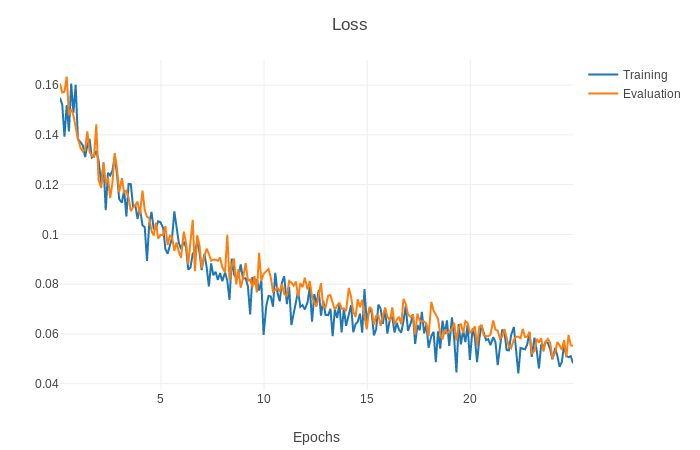Out: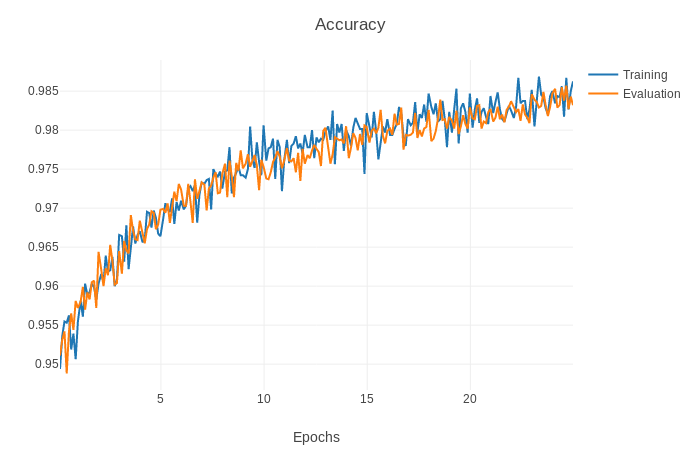## Summary and Next Steps¶

In this post, you've learnt how to:

• augment image data with transforms;
• normalise inputs;
• use activation functions;
• use dropout;
• train and evaluate a Convolutional Neural Network.

In the next post, we'll be looking at how to use a pre-trained network to create a custom object recogniser.

## Acknowledgements¶

This post has been inspired by posts from: